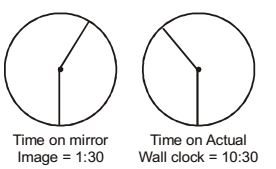2,976 views

Two and quarter hours back, when seen in a mirror, the reflection of a wall clock without number markings seemed to show $1:30$. What is the actual current time shown by the clock?

1. $8:15$
2. $11:15$
3. $12:15$
4. $12:45$

Reflection of time is 1:30

so actual time is ( 11:60  -   1:30 ) = 10:30

here mentioned 2:15 hrs back

so add it to 10:30

we got  12:45

the actual current time shown by the clock is 12:45

Correct Answer: $D$

Mirror reflection = 12:00 - actual time(12 numbers in a clock)

12:00 is same as 11 hrs 60 min. So, 11:60-1:30
Is it digital clock or analog clock ? If it is digital clock then how to solve it?
Yes bro! in digital clock we can see needles , right ? :)So present time = 10:30 + 2:15 = 12:45

Correct option : D

by